Select PageDonald R. Van Deventer, Ph.D.

Don founded Kamakura Corporation in April 1990 and currently serves as its chairman and chief executive officer where he focuses on enterprise wide risk management and modern credit risk technology. His primary financial consulting and research interests involve the practical application of leading edge financial theory to solve critical financial risk management problems. Don was elected to the 50 member RISK Magazine Hall of Fame in 2002 for his work at Kamakura.

A 15-Factor Heath, Jarrow, and Morton Stochastic Volatility Model for the German Bund Yield Curve, Using Daily Data from August 7, 1997 through September 30, 2021

10/06/2021 10:44 AM

Donald R. van Deventer

First Version: October 6, 2021

This Version: October 6, 2021

ABSTRACT

Please note: Kamakura Corporation term structure models are updated monthly.  For the most recent set of coefficients, contact info@kamakuraco.com

This paper analyzes the number and the nature of factors driving the movements in the German government (“Bund”) yield curve from August 7, 1997 through September 30, 2021. The process of model implementation reveals a number of important insights for interest rate modeling generally. First, model validation of historical yields is important because those yields are the product of a third-party curve fitting process that may produce spurious indications of interest rate volatility. Second, quantitative measures of smoothness and international comparisons of smoothness provide a basis for measuring the quality of simulated yield curves. Third, we outline a process for incorporating insights from the Japanese and European experience with negative interest rates into term structure models with stochastic volatility in Germany and other countries.  Fourth, we compare data availability for Germany with broad international experience to measure the risk that a simulation beyond historical rate levels in Germany could go awry. Finally, we illustrate the process for comparing stochastic volatility and affine models of the term structure.  We conclude that stochastic volatility models have a superior fit to the history of yield movements in the German Bund market. We also recommend that Germany Bund interest rate risk analysis employ the full “World” 13-country term structure model rather than relying solely on German data alone.

A 15-Factor Heath, Jarrow, and Morton Model

for the German Bund Yield Curve,

Using Daily Data from August 7, 1997 through September 30, 2021

Government yield curves are a critical input to the risk management calculations of central banks, bank regulators, major banks, insurance firms, fund managers, pension funds, and endowments around the world.  With the internationalization of fixed income investing, it is important to understand the dynamics of movements in yield curves world-wide, in addition to the major bond markets like those in Frankfurt, London, New York and Tokyo. In this paper, we fit a multi-factor Heath, Jarrow and Morton model to daily data from the German Bund market over the period from August 7, 1997 to September 30, 2021. The modeling process reveals a number of important implications for term structure modeling in other government bond markets.

Section I discusses the origin and characteristics of the daily data base of German Bund yields provided by the Deutsche Bundesbank.  We discuss yield curve smoothness and volatility fitting as measures for judging the quality of government-generated yield curve time series, including German Bund yield curves.  We conclude that the Deutsche Bundesbank time series is realistically smooth and a reliable foundation for term structure modeling. This compares with recent findings from Australia, Japan, and Thailand where we found that yield curves were unrealistically rough and that modification of the input data was necessary for a realistic model, a standard part of a Bayesian model validation process.

Section II outlines the process for determining whether the interest rate volatility for the factors driving the German Bund yield curve is constant (i.e., non-random, an “affine” model) or stochastic, typically expressed as a function of the level of interest rates.  We note the extensive experience with negative interest rates in the European and Japanese government bond markets and use insights from that experience in fitting volatility in the German Bund market, where negative rate experience has been extensive. Section III describes the process of fitting five different Heath, Jarrow, and Morton models to German Bund yield data: models with 1, 2, 3, 6 and 15 factors. We conclude Section III with Bayesian model validation procedures based on stress tests of the derived volatility and drift functions. Section IV concludes the paper.   Appendix A illustrates a sample model validation process for widely used one-factor term structure models. Appendix B includes additional analysis of empirical drift and stress tests of rate volatility and compares German results to the 13-country World term structure model.

I. German Bund Data: Special Characteristics

A multi-factor term structure model is the foundation for best practice asset and liability management, market risk, economic capital, interest rate risk in the banking book, stress-testing, and the internal capital adequacy assessment process.  The objective in this paper is to illustrate the derivation of a multi-factor Heath Jarrow and Morton model of the German Bund yield curve.  As a by-product, the analysis has the potential to detect common data problems associated with yield curve histories and employs a standard methodology for quantification and resolution of those problems. Previous implementations of multi-factor Heath, Jarrow and Morton models have covered the following bond market sectors:

• Australia                   Commonwealth Government Securities
• France                      French Government Securities
• Germany                  German Bunds (prior version)
• Italy                          Italian Government Securities
• Japan                       Japanese Government Bonds
• Russia                      Russia Government Securities
• Singapore                Singapore Government Securities
• Spain                        Spanish Government Bonds
• Sweden                    Swedish Government Securities
• Thailand                   Thai Government Securities
• United Kingdom       United Kingdom Government Bonds
• United States           Treasury Securities

The first step in data model validation for the German Bund market is to examine the historical availability of bond yields over time.  This availability is summarized in Table I.

Table IThe data shows that the German Bund data history is atypical in that the Deutsche Bundesbank time series shows no changes in “data regime,” i.e., which of the maturities are available on a given date.  Canadian and Russia government yields provide other examples of a single data regime.

Because our Heath, Jarrow and Morton analysis makes use of a yield curve with 91-day (quarterly) forward rate segments, the next step in data model validation is to fit quarterly forward rates to the raw coupon-bearing bond yields.  The smoothness of the resulting forward rates will be a function of both the quality of the raw data from a smoothness point of view and the smoothness implied by the secondary smoothing process.  To ensure the maximum smoothness from the secondary smoothing process, we use the maximum smoothness forward rate methodology of Adams and van Deventer , as corrected in van Deventer and Imai . Adams and van Deventer show that the maximum smoothness method overcomes the problems of the cubic spline approach of McCulloch, and, unlike the Svensson  approach, allows for a perfect fit to the raw data provided by the Deutsche Bundesbank.  See Jarrow  for information on the problems with Svensson yield curve fitting.

We then conduct a visual inspection of the resulting forward rates implied by the raw data. The smoothness of the quarterly forward rate curve can be measured quantitatively using the quarterly forward rates implied by the German Bund yield curves.  For a yield curve that consists of N quarterly forward rates, the discrete smoothness statistic at time t, ZN(t), is the sum of the squared second differences in the forward rates, as explained by Adams and van Deventer. A closed form continuous smoothing statistic can also be calculated when the functional form of the continuous forward rate is known (as it is in this case).  The discrete smoothness statistic is given here: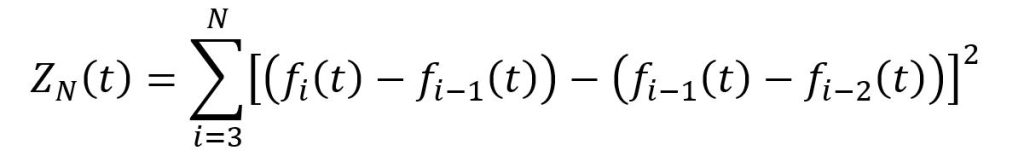The German Bund series is realistically smooth, and we use that data with confidence in what follows.  We apply a final screen for outliers in the process of deriving the stochastic volatility functions.  Yield curves on the following days were omitted from estimation because they implied implausible forward rate shocks in selected 91-day forward rates:

• May 2, 2003
• August 1, 2003
• September 30, 2008
• October 1, 2008
• October 9, 2008
• October 10, 2008
• December 30, 2008
• January 8, 2009
• January 9, 2009

II. Constant versus Stochastic Volatility

Constant volatility (“affine”) term structure models are commonly used for their ease of simulation and estimation of “future expected rates” in order to determine the “term premium” in current yields.  Prominent examples are Adrian, Crump and Moench , Kim and Wright , and Duffie and Kan . On the other hand, the weight of the empirical evidence in most of the countries studied to date indicates that interest rate volatility does vary by the level of the corresponding forward rate.  To illustrate that fact, we studied the shortest forward rate on the German Bund yield curve on a daily basis from August 7, 1997 through September 30, 2021.  We ordered the data from lowest forward rate level to highest forward rate level.  We formed non-overlapping groups using the larger of 50 observations or 1/100th of total observations each and calculated both the standard deviation of 91-day forward rate changes and the mean beginning-of-period forward rate in each group.  The results are plotted in Exhibit III:

Exhibit III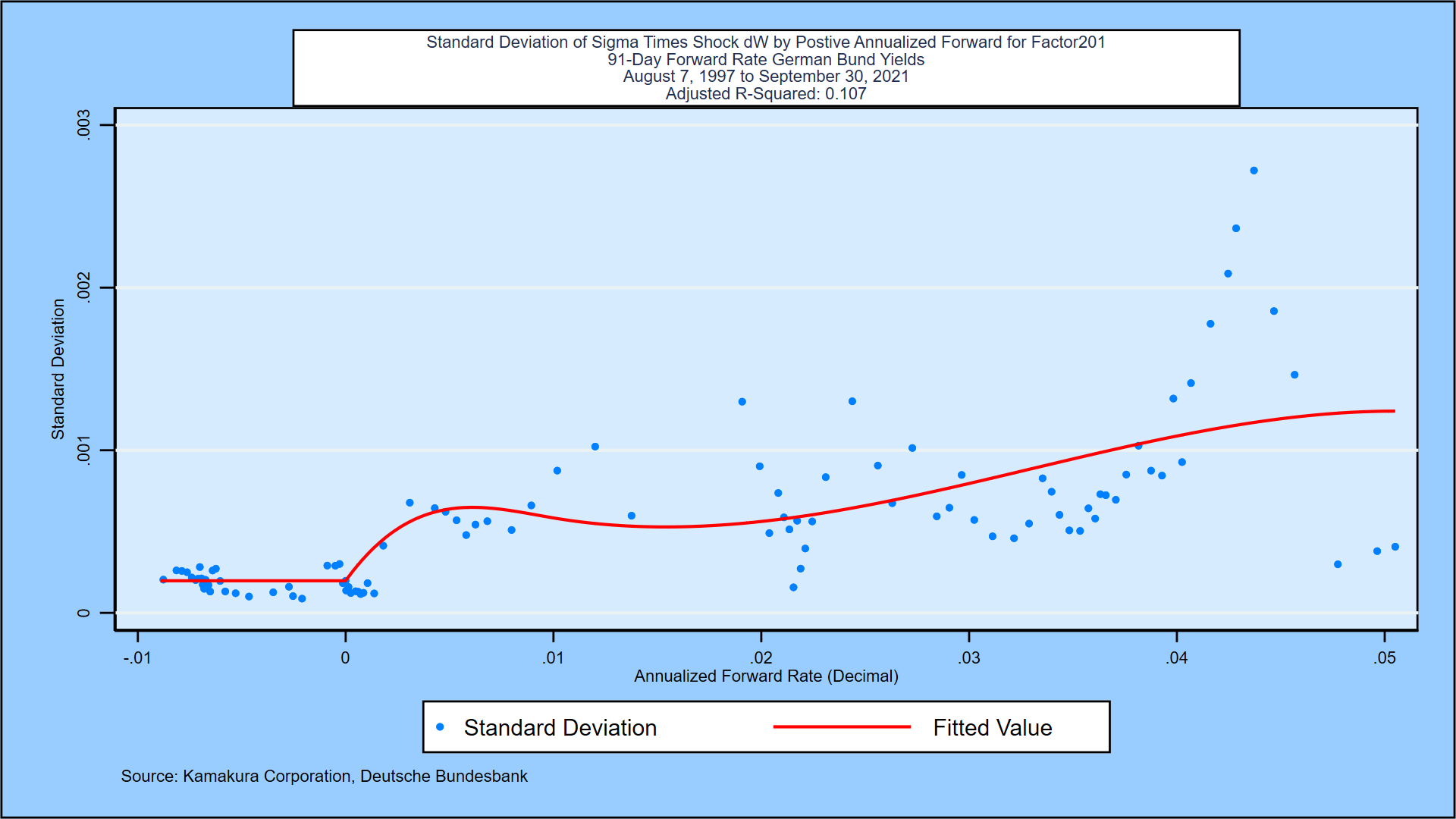A 2-segment cubic spline of annualized forward rates, combined with a constant volatility when rates are negative, explains only 10.7% of the variation in the standard deviation of forward rate changes for these ordered groups. This is the volatility function used when extracting the first random factor driving the German Bund yield curve. Note that the right-hand side of the curve has been constrained to have a first derivative of zero at a high level of rates. The rise in volatility in higher rate environments has been confirmed in the government securities markets for Australia, Canada, France, Germany, Italy, Japan, Russia, Singapore, Spain, Sweden, Thailand, the United Kingdom, and the United States.  Exhibit IV shows the results for the second risk factor in the German Bund market, the idiosyncratic movements in the quarterly forward rate maturing in 30 years:

Exhibit IVThe cubic spline stochastic volatility specification explains 84.5% of the observed variation in forward rate volatility in the quarterly forward rate maturing at the 30-year point on the German Bund yield curve.  We have imposed the same constraint on the first derivative and require that the fitted volatility not be less than the lowest observed volatility, which we discuss later in this section.  The unusual and relatively poor fit of volatility to rate levels for Factor 1 is one of the reasons why we suggest later in the paper that the 13-country World model adds to the robustness of term structure model parameters for the German Bund HJM model.

Exhibit V shows the historical movements in German Bund zero-coupon yields over the historical period studied.  In later sections, we compare the span of the German data set with the range of rates and maturities contained in the 13-country World model.  The German data set spans 42.32% of the World experience, arrayed by rate level and years to maturity.  This is of great importance and we return to this topic in depth below.

Exhibit VExhibit VI below shows the evolution of the first quarterly forward rate (the forward that applies from the 91st day through the 182nd day) over the same time period:

Exhibit VIWe use three statistical tests to determine whether or not the hypothesis of normality for forward rates and zero-coupon bond yields should be rejected at the 5% level: the Shapiro-Wilk test, the Shapiro-Francia test, and the skew test, all of which are available in common statistical packages. The German data history is over the maximum number of observations allowed by these tests.  The hypothesis of normality was rejected at each 91-day forward rate maturity date when testing earlier samples with a smaller observation count.

The rejection of the hypothesis of normality is problematic for constant (more precisely, non-random) coefficient or “affine” term structure models. In all of the other countries studied, the hypothesis of normality has been rejected strongly as well.  Given these results, we proceed with caution on the implementation of the affine model.

In Chapter 3 of Advanced Financial Management (second edition, 2013), van Deventer, Imai and Mesler analyze the frequency with which forward rates move up together, down together or remain unchanged. This exercise informs the Heath, Jarrow and Morton parameter fitting process and is helpful for the model validation questions posed in the Appendix. We perform the yield curve shift analysis using 6,058 days of quarterly forward rates for the German Bund yield curve. We analyze the daily shifts in the forward rates on each business day from August 7, 1997 through September 30, 2021. The results are given in Table II:

Table IIYield curve shifts were all positive, all negative, or all zero 7.94%, 8.58%, and 2.49% of the time, a total of 19.02% of all business days.  The predominant yield curve shift was a twist, with a mix of positive changes, negative changes, or zero changes.  These figures are similar to those for the 12 other countries for which we estimate term structure model parameters on a regular basis. These twists, which happen 80.98% of the time in Germany, cannot be modeled accurately with the conventional implementation of one-factor term structure models.

Another important aspect of yield curves is the number of local minima and maxima that have occurred over the modeling period.  The results for the German Bund market are given in Table III:

Table IIIThe number of days with 0 or 1 humps (defined as the sum of local minima and maxima on that day’s yield curve) was 74.55% of the total observations in the data set.

Finally, before proceeding, we count the number of occurrences of negative rates for each forward rate segment of the yield curve over the history provided by the Deutsche Bundesbank and report on the observed 91-day volatility of forward rates when the start of the period annualized forward rate is negative, zero, and positive.

Table IV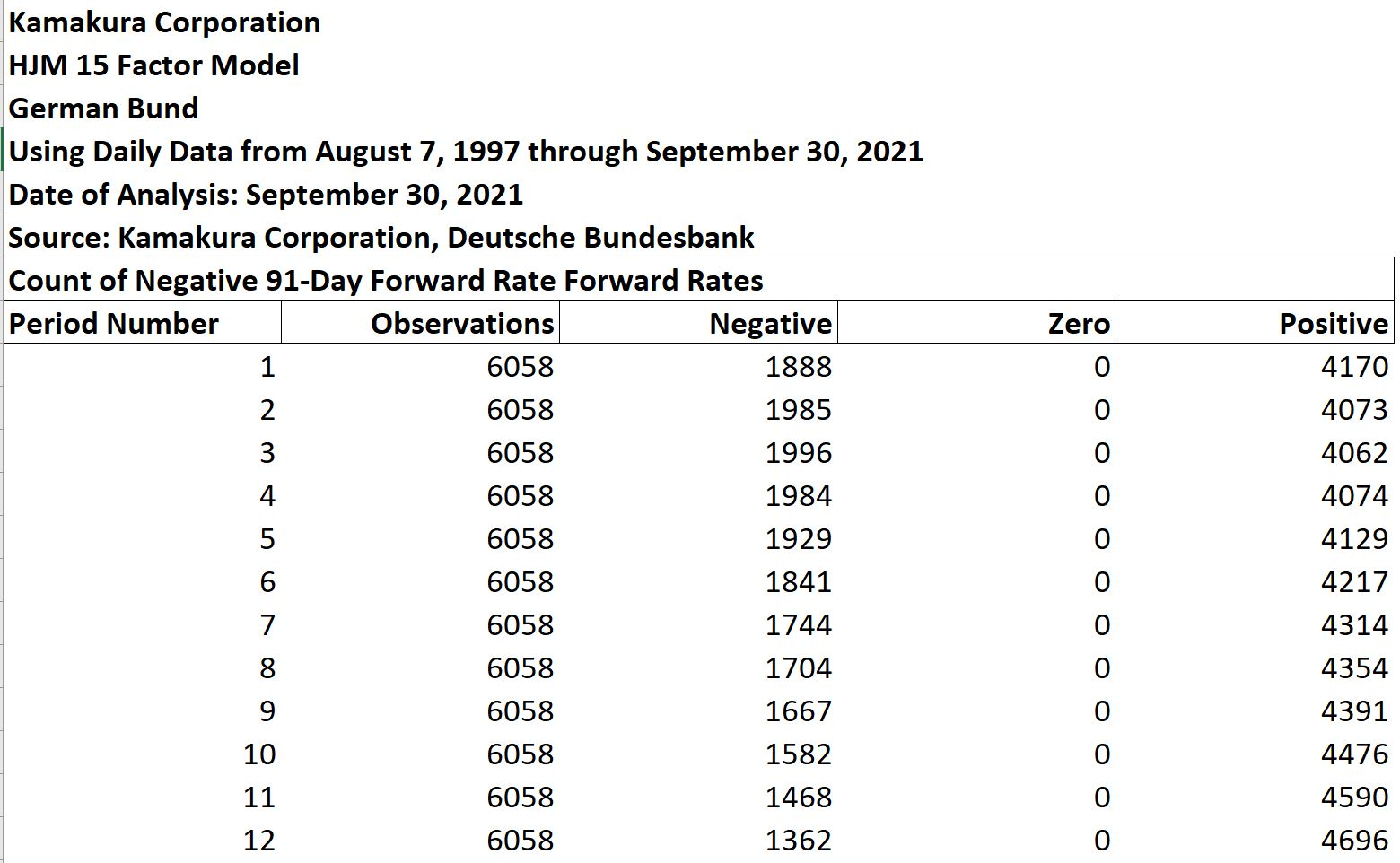The German Bund yield curves imply a very large number of negative rates in the first 12 91-day periods analyzed.  Out of the 6,058 total observations, the number of negative observations ranges from a low of 1,362 at the 12th forward rate to a high of 1,996 for the 3rd forward rate.

The next exhibit shows that negative rates at the 1-month time horizon have become common among the 13-country World interest rate data base maintained by Kamakura Corporation.  Of 90,814 observations at a 1-month maturity, 12.044% of World experience has been negative.

Exhibit VII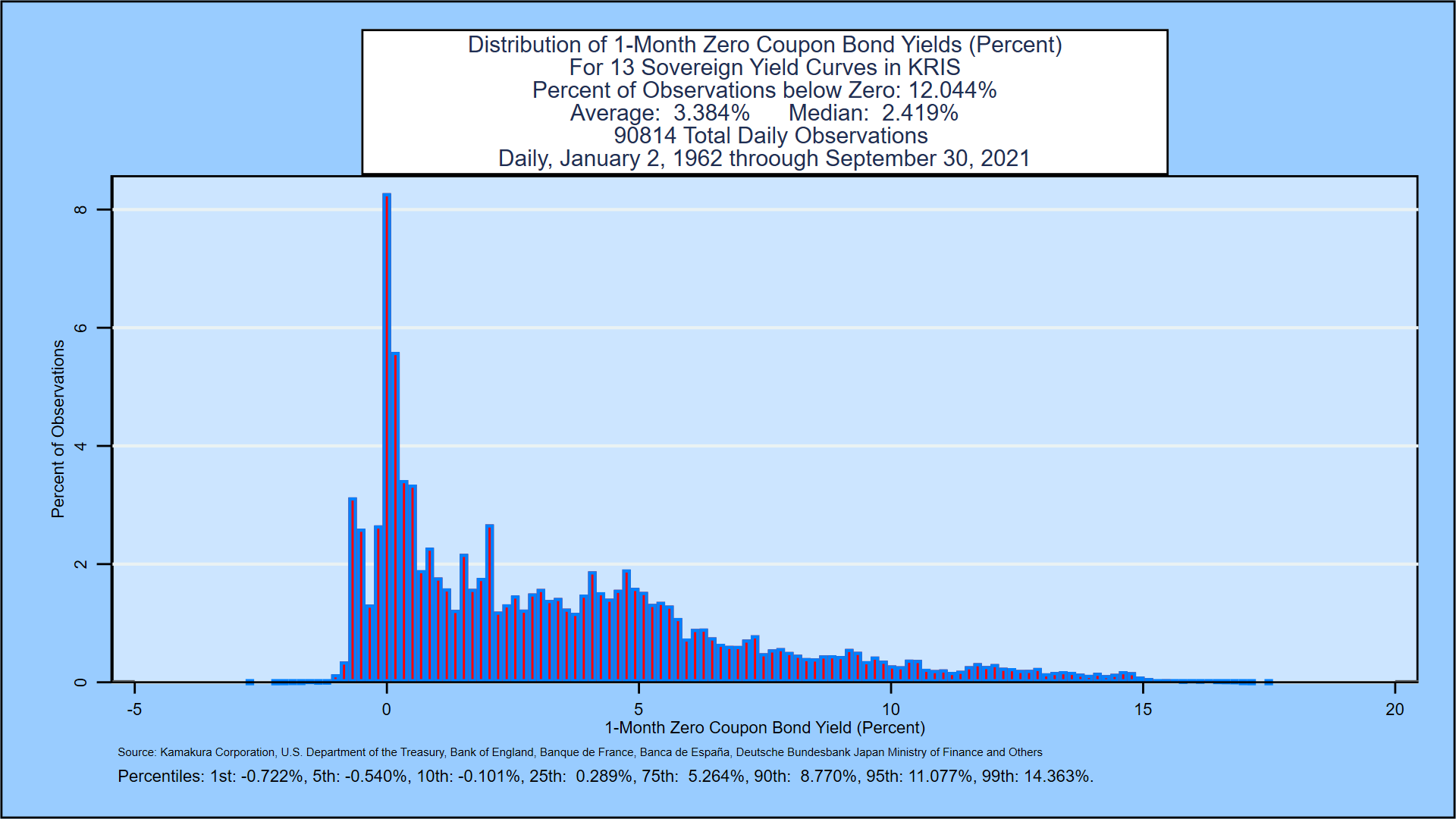Observed volatility in 1-month zero-coupon bond yields as a function of interest rate level for the standard 13-country World data set is shown in Exhibit VII:

Exhibit VIIIThree key observations stand out: volatility is not constant across rate levels, volatility is never zero, even at negative rate levels, and volatility trends upward as rate levels rise.

III. Fitting Heath, Jarrow, and Morton Parameters

A simple first step in constructing a multi-factor Heath, Jarrow and Morton model is to conduct principal components analysis on the forward rates that make up the relevant yield curve.  For the German Bund market, at its longest maturity, these quarterly segments consist of one three-month spot rate and 119 forward rates.  Over 5,766 observations, the principal components analysis indicates in Table V that the first factor explains 74.01% of the movement in forward rates over the full curve. This is a higher percentage than average for the other 12 countries in the World model. For a high degree of explanatory power, the principal components analysis indicates that 6 to 16 factors will be necessary.

Table VWith this analysis as background, we begin the Heath, Jarrow, and Morton fitting process.

In the studies done so far, the number of statistically significant factors is summarized below:

• Australia:                  Commonwealth Government Securities, 14 factors
• France:                     French Government Bonds, 7 factors
• Germany:                 Bunds, 14 factors (prior version)
• Italy:                         Italian Government Bonds, 11 factors
• Japan:                      Japanese Government Bonds,  8 factors
• Russia:                     Russia Government Bonds, 11 factors
• Singapore:               Singapore Government Securities, 9 factors
• Spain:                      Spanish Government Securities, 11 factors
• Sweden:                   Swedish Government Securities, 11 factors
• Thailand:                  Thai Government Securities, 11 factors
• United Kingdom:      Government Securities, 14 factors
• United States:          U.S. Treasury Bonds, 10 factors
• World:                      13 countries’ government securities,12 factors

We now fit a multi-factor Heath, Jarrow and Morton model to German Bund zero-coupon yield data from August 7, 1997 to September 30, 2021. The procedures used to derive the parameters of a Heath, Jarrow and Morton model are described in detail in Jarrow and van Deventer (June 16, 2015 and May 5, 2017).

We followed these steps to estimate the parameters of the model:

• We extract the zero-coupon yields and zero-coupon bond prices for all quarterly maturities out to 30 years for all daily observations for which the 30-year zero-coupon yield is available. This is done using Kamakura Risk Manager, version 10.1, using the maximum smoothness forward rate approach to fill the quarterly maturity gaps in the zero-coupon bond data.
• We use overlapping 91-day intervals to measure changes in forward rates, avoiding the use of “quarterly” data because of the unequal lengths of calendar quarters. Because overlapping observations trigger auto-correlation, “HAC” (heteroscedasticity and autocorrelation consistent) standard errors are used. The methodology is that of Newey-West with 91-day lags.
• We consider 15 potential explanatory factors: the idiosyncratic portion of the movements in quarterly forward rates that mature at the 15 yield curve maturities given below.
• We calculate the discrete changes in forward returns as described in the parameter-fitting technical guide. Because the discrete changes are non-linear in the no-arbitrage framework of Heath, Jarrow, and Morton, we use generalized linear models and the proper HJM exponential functional form to fit interest rate volatility.
• We use a different non-linear regression for each segment of the yield curve. We considered a panel-based approach, but we rejected it for two reasons: first, the movement of parameters as maturity lengthens is complex and not easily predictable before estimation; second, the residual unexplained error in forward rates is very, very small, so the incremental merit of the panel approach is minimal.
• We then begin the process of creating the orthogonalized risk factors that drive interest rates using the Gram-Schmidt procedure. Without loss of generality, these factors are assumed to be uncorrelated independent random variables that have a normal distribution with mean zero and standard deviation of 1.
• Because interest volatility is assumed to be stochastic, simulated out-of-sample forward rates will not in general be normally distributed. We also calculate constant volatility parameters and choose the most accurate from the constant volatility and stochastic volatility models estimated.
• In the estimation process, we added factors to the model as long as each new factor provided incremental explanatory power. The standard suite of models in both cases includes 1 factor, 2 factors, 3 factors, 6 factors and “all factors,” which varies by country.

We postulate that the unannualized interest rate volatility for each 91-day forward rate maturity k is a cubic function of the annualized forward rate that prevails for the relevant risk factor j at the beginning of each 91-day period:When the initial forward rate is negative, we postulate that interest rate volatility is a constant. Using Japan volatility data reported above, the measured b0,jk was 0.1408%. Using the 13-country World model, the value of b0,jk was 0.0478%.

We use the resulting parameters and accuracy tests to address the hypothesis that a one-factor model is “good enough” for modeling German Bund yields in Appendix A. We report the accuracy results for 1, 2, 3, 6 and all (15) factors.  The factors are the idiosyncratic variation in quarterly forward rates at each of 15 maturities.  The factors, described by the maturity of the 91-day forward rate used, are added to the model in this order:

Data Regime 1 (Maturities of 30 years or less)

• Factor 1:       6 months
• Factor 2:       30 years
• Factor 3:       5 years
• Factor 4:       10 years
• Factor 5:       2 years
• Factor 6:       20 years
• Factor 7:       7 years
• Factor 8:       15 years
• Factor 9:       1 year
• Factor 10:     25 years
• Factor 11:     8 years
• Factor 12:     3 years
• Factor 13:     9 years
• Factor 14:     4 years
• Factor 15:     6 years

Exhibit IX summarizes the adjusted r-squared for the non-linear equations for each of the 119 quarterly forward rate segments that make up the German Bund yield curve:

Exhibit IXThe adjusted r-squared for the best practice model over each of the forward rates is plotted in blue and is near 100% for all 119 quarterly segments of the yield curve.  The one-factor model in red, by contrast, does a poor job of fitting 91-day movements in the quarterly forward rates.  The adjusted r-squared is good, of course, for the first forward rate since the short rate is the standard risk factor in a one-factor term structure model.  Beyond the first quarter, however, the explanatory power of a one-factor model declines rapidly.  The adjusted r-squared of the one-factor model never exceeds 20% after the first 17 quarterly forward rates and is below that level at most maturities.

The root mean squared error for the 1, 2, 3, 6 and all (15) factor stochastic volatility model is shown in Exhibit X.

Exhibit X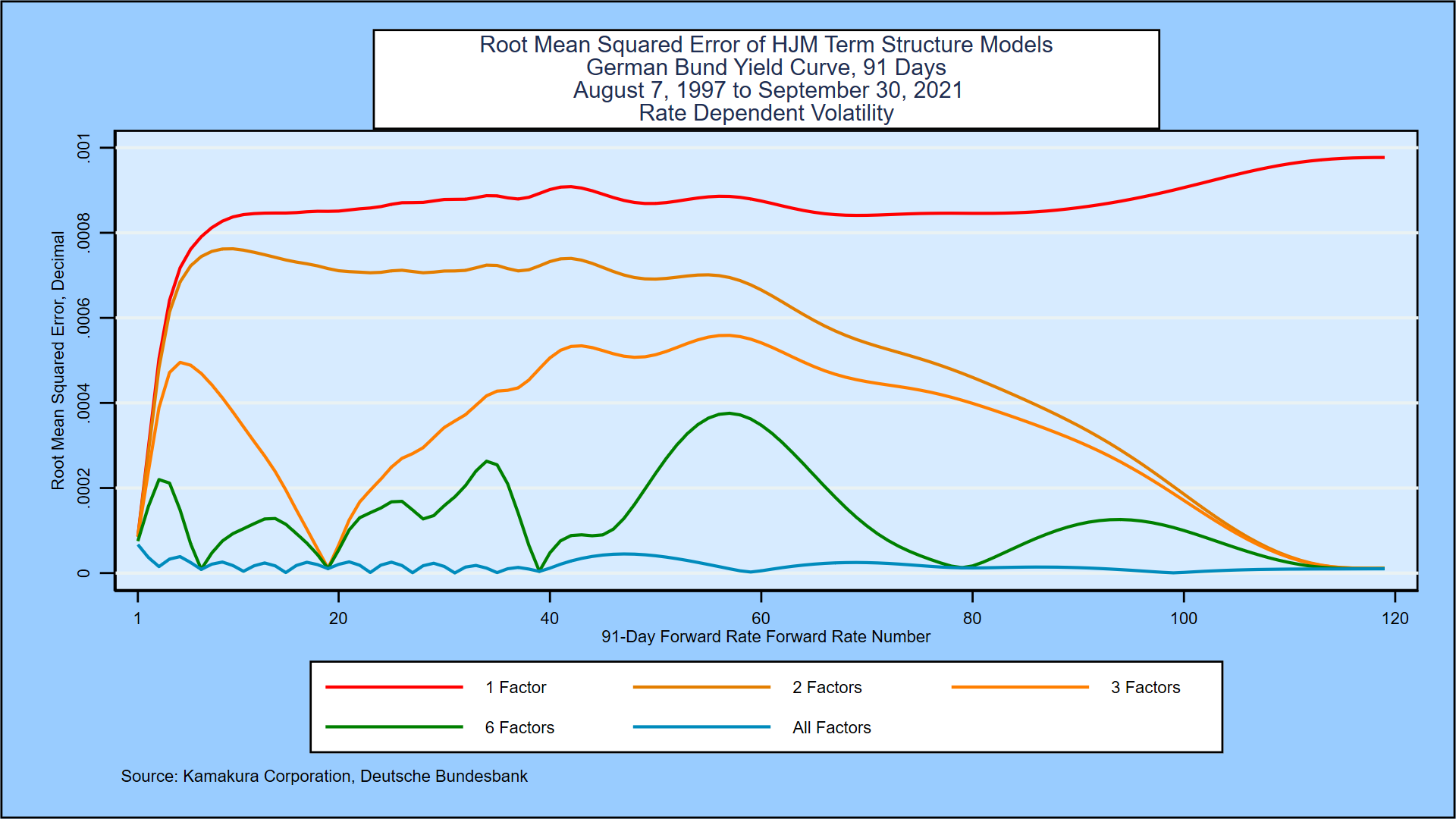The root mean squared error for the 15-factor model is less than 0.01% at every maturity along the yield curve. This result should not come as a surprise to a serious analyst, because it is very similar to the results of the best practice Heath, Jarrow and Morton term structure models for the other 12 government bond markets studied.

Bayesian Considerations in Model Validation

Kamakura term structure model validation is conducted in the spirit of Bayesian iterative model building as outlined by Gelman et al .  This quote from Gelman et al  explains the Bayesian estimation process:

“The process of Bayesian data analysis can be idealized by dividing it into the following three steps:

1. Setting up a full probability model—a joint probability distribution for all observable and unobservable quantities in a problem. The model should be consistent with knowledge about the underlying scientific problem and the data collection process.
2. Conditioning on the observed data: calculating and interpreting the appropriate posterior distribution—the conditional probability distribution of the unobserved quantities of ultimate interest, given the observed data.
3. Evaluating the fit of the model and the implications of the resulting posterior distribution: how well does the model fit the data, are the substantive conclusions reasonable, and how sensitive are the results to the modeling assumptions in step 1? In response, one can alter or expand the model and repeat the three steps.”

Jarrow and van Deventer go on to explain that the iterative process described above by Gelman et al is especially important in fitting Heath, Jarrow, and Morton parameters for the following reasons:

1. Negative interest rates have been observed in Japan, Hong Kong, Germany, and many other European countries, but many other countries, including the U.S. and Russia, have yet to report negative rates on central bank websites. In the U.S. case, the U.S. Department of the Treasury notes on its website that it overrides observed negative yields in the market with zero values.
2. The “knowledge about the underlying scientific problem” from the historical data available is as follows: (1) negative rates are possible, (2) they are less likely to occur than positive rates, (3) interest rate volatility that results when rates are negative is of high interest but the historical data is still limited or non-existent, depending on the country, and (4) an international data set would best shed light on this and other HJM issues.

There are other issues relevant to estimation:

1. As noted by Heath, Jarrow, and Morton , stochastic volatility driven by interest rate levels must be capped to avoid a positive probability of infinitely high interest rates.
2. Subject to this cap, most market participants expect interest rate volatility to rise as rates rise and that the interest rate volatility that prevails when rates are negative probably represents the lowest level of volatility that would prevail. Historical experience with negative rates so far around the world makes it clear that interest rate volatility does not go to zero at any rate level.
3. Most market participants believe that the empirical drift in forward rates that occurs (i.e., the change in observed empirical interest rates in the case where all interest rate shocks are zero) varies by the level of interest rates. The stochastic volatility model described in this paper assumes that empirical drift is a cubic function of annualized forward rates.  The results show the expected mean reversion, which we discuss in Appendix B.

To summarize, a model validation effort in the Bayesian spirit would address at least these issues:

• Tests of smoothness of simulated curves
• Tests to confirm existence of negative rates in selected circumstances in the simulation
• Comparison of simulated risk neutral and empirical yields
• Time series distribution of simulated risk neutral and empirical yields
• Tests for the reasonableness of empirical drift in rates at each segment of the yield curve and stress tests of the rate factor volatility functions for reasonableness over the full range of rates that have been observed in the past. These tests are shown in Appendix B

We can conduct an inspection of these issues with the aid of a forward-looking out-of-sample simulation of German Bund yields with the following specifications:

• Yield curve: German Bund yields
• Date of yields: Most recent date
• Number of scenarios: 500,000 or more
• Simulation time horizon: Longest observable yield available (30 years in Germany)
• Simulation periodicity: 91 days (quarterly)

Smoothness Validation

In the simulation, we select a random sample of 10 scenarios at each time step and visually examine forward rate graphics for smoothness.  We can also use the discrete formula for smoothness given above to identify any outliers and examine the scenarios in question.

These graphs provide informal confirmation that nothing in the model estimation procedure has introduced artificial lumpiness in the simulated yield curves.  A quantitative assessment of the smoothness of all 500,000 yield curves at each time step would provide the more formal confirmation that the yield curves simulated are realistically smooth.

Distribution of Simulated Risk Neutral and Empirical Rates

We then examine the probability distributions of risk neutral and empirical simulated rates at various maturities over time.  We seek to detect visually any points in time at which the simulated distribution of yields is strange or unrealistic.

We seek to determine that the simulation is reasonable from multiple dimensions.  Rates can be negative but (for empirical yields) the probability of negative rates is relatively low.  On the long end of the maturity spectrum, we usually find that rates do rise to the 20% range but with a very low probability.

Time Series Distribution of Simulated Yields

We also plot the time series graphs of the mean, median, high, low, and various percentiles for empirical rates.  We again seek to determine that there are no unexpected variations in the distribution of empirical yields over time.

The size of the “term premium” of actual zero-coupon yields over the expected level of the short rate is a topic of great interest to both academics and policy makers.  In a stochastic volatility model, the term premium must be determined by simulation because in general there is no closed form solution for expected future rates.  We again reach conclusions about whether the simulation produces results that are consistent with the “scientific knowledge” about the variation in interest rates around the world.

Probability of Negative Short-term Interest Rates

We also seek to determine whether or not there is a realistic possibility of negative rates in the simulation.

Comparing German Data with World Experience

A common econometric challenge is fitting a model to the data at hand and measuring the risk that the model behaves badly out of sample.  One measure of this risk for the German data set is to compare the range of interest rates experienced in Germany with the 13-country World data set over maturities out to 30 years.  We use zero-coupon bond yields in a common format across countries and maturities for these calculations.

The following Exhibit XI shows that, measured by maturity range and interest rate range, German data spans 42.32% of World experience:

Exhibit XIAdditional stress tests for empirical drift of rates and for interest rate volatility reported in Appendix B confirm what this exhibit implies: for a realistic German Bund interest rate simulation that performs well “out of sample” (compared to the Bund yield history), the best term structure model to use would be the World model rooted in the experience of 13 countries, including Germany.  This conclusion applies to all but one or two countries (the U.S. and the United Kingdom).  Even in the U.S. case, the lack of experience with negative rates means the World model is likely to produce best results.

IV. Conclusion

The Germany Bund yield curve is driven by 15 factors, a number of factors that is above the average of what we have found for government yield curves in 12 other markets for which studies have been conducted.  The August 7, 1997 to September 30, 2021 yield history for Germany Bunds is fairly typical, but the lack of experience with very high rates results in a sparser data environment than most analysts would want for a robust stand-alone single country term structure model.

The stochastic volatility assumption provides more accurate and more reasonable parameters than a constant volatility model, particularly in the context of Bayesian simulations as part of the model validation process.  Exhibit XII summarizes the reasons for those conclusions:

Exhibit XII: Statistical Significance Summary and Volatility Classification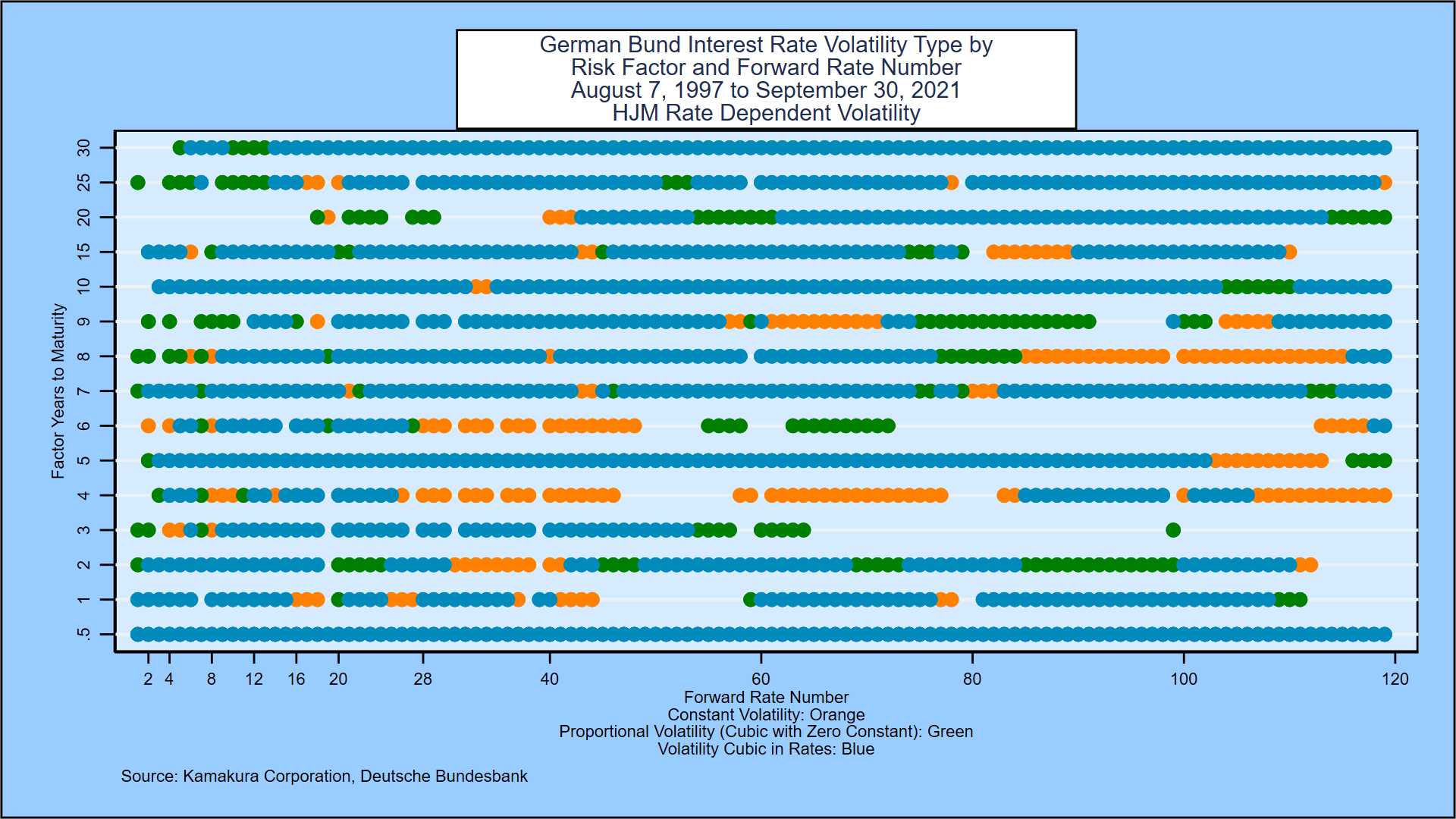The vertical axis lists the maturities used as risk factors by years to maturity of the underlying quarterly forward rate. The risk factors are the idiosyncratic movement of each of these forward rates.  If the risk factor is statistically significant in explaining the movement of forward rates with the quarterly maturities listed on the horizontal axis, a dot is placed in the grid.

The nature of interest rate volatility for each combination of risk factor maturity and forward rate maturity is color coded.  If the derived volatility is constant, the color code is orange.  This is the affine specification.  The graph shows immediately that only a small minority of the risk factor maturity/forward rate maturity volatilities are consistent with the affine structure (the orange dots). The green and blue codes address the issue of whether interest rate volatility for that combination of risk factor maturity and forward rate maturity is zero or not when the forward rate is zero.  If the measured volatility at a zero forward rate level is zero, the color code is green.  Otherwise, the color code is blue.  In both cases, the volatility is a stochastic function of the forward rates at the start of the simulation period.

The chart summarizes the fact that all 15 factors are statistically significant across the yield curve for German Bunds.  The dominant derived interest rate volatility is the cubic stochastic volatility specification with a non-zero constant.  An affine assumption for interest rate volatility is best fitting for a very small minority of the combinations of risk factor maturity and forward rate maturity.

That being said, Exhibit XIII shows that the average root mean squared error for a 15-factor constant coefficient model is slightly below the average root mean squared error for the stochastic volatility model.  Additional research on this point is forthcoming in subsequent editions of this paper.

Exhibit XIII: Root Mean Squared Error ComparisonAppendix A: Single-Factor versus Multiple-Factor Models

Despite the overwhelming evidence across countries that government bond yields are driven by multiple factors, the use of single-factor term structure models in interest rate risk management systems remains common even in some of the world’s largest financial institutions.  This appendix asks and answers a number of important questions on the use of one-factor models that any sophisticated model audit would pose.  Given the answers below, most analysts would conclude that one-factor term structure models are less accurate than a long list of multi-factor term structure models and that the one-factor models would therefore fail a model audit.

We address two classes of one-factor term structure models, all of which are special cases of the Heath, Jarrow, and Morton framework, in this appendix using data from the German Bund market and the World data set. Answers for other government bond markets cited in the references are nearly identical.

One-factor models with rate-dependent interest rate volatility:

• Cox, Ingersoll, and Ross (1985)
• Black, Derman and Toy (1990)
• Black and Karasinski (1991)

One-factor models with constant interest rate volatility (affine models):

• Vasicek (1977)
• Ho and Lee (1986)
• Extended Vasicek or Hull and White Model (1990, 1993)

Non-parametric test 1: Can interest rates be negative in the model?

The one-factor models with rate-dependent interest rate volatility make it impossible for interest rates to be negative without ad hoc shifts in the simulated yield curves. Is this implication true or false? It is false, as this histogram of 1-year zero-coupon bond yields from government securities in 13 countries confirms. 10.280% of observations were negative:

Exhibit A1At a 1-month maturity, the percentage of negative rates is 12.044%:

Exhibit A2Non-parametric test 2: As commonly implemented, one-factor term structure models imply that all yields will either (a) rise, (b) fall, or (c) remain unchanged.  This implication is false, as documented for the German Bund yield curve in Table II.  In fact, yield curves have twisted on 80.98% of the observation dates for the German Bund market.

Non-parametric test 3: The constant coefficient one-factor models imply that zero-coupon yields are normally distributed and so are the changes in zero-coupon yields.  For all markets tested, this implication is rejected by three common statistical tests for all of the quarterly maturities for zero yields and for all of the quarterly changes.  This histogram (shown above) of 1-month zero-coupon bond yields from government bond markets in 13 countries provides visual confirmation that normality is a poor approximation to the probability distribution of interest rates.

Assertion A: There are no factors other than the short-term rate of interest that are statistically significant in explaining yield curve movements.  This assertion is false. Table VI shows, using principal components analysis, that 6-16 factors are needed to explain the movements of the German Bund yield curve.  Exhibits IX and XII make the same point in more detail.

Assertion B: There may be more than one factor, but the incremental explanatory power of the 2nd and other factors is so miniscule as to be useless.  This assertion is false, as the 2nd through 15th factors in the German Bund market explain 25.99% of forward rate movements, compared to 74.01%  for the first factor alone.  In most countries, the best “first factor” is not the short rate of interest used by many large banks; it is the parallel shift factor of the Ho and Lee model.

Assertion C: A one-factor “regime shift” model is all that is necessary to match the explanatory power of the 2nd and other factors.  This assertion is also false.  A recent study prepared for a major U.S. bank regulator also confirmed that a one-factor “regime shift” term structure model made essentially no incremental contribution toward resolving the persistent lack of accuracy in one-factor term structure models.

Finally, a Kamakura Corporation study comparing 1-factor and 10-factor Heath Jarrow and Morton simulations for the U.S. Treasury curve shows that the simulated volatility of interest rates is underestimated by 61% to 83% in the 1-factor model.  Moreover, the average level of simulated yields is biased lower.  Finally, the probability of negative rates implied by the 1-factor model is also biased low as shown below:

Table A1Appendix B: Tests of Empirical Drift and Stress Tests of Rate Volatility

Many of the Bayesian confirmations that out-of-sample simulations will be “consistent with our scientific knowledge of the problem” can in fact be gleaned without a full simulation. One practical modeling objective is to obtain out-of-sample rate scenarios that “do not blow up,” rising or falling to extreme values that are highly unlikely to occur in reality.  Heath, Jarrow, and Morton make it clear how this objective is achieved in an HJM model:

1. First of all, rate shocks and rate volatilities are the same for the simulation of both risk-neutral and empirical rates.
2. This requires that the interest rate volatility functions be capped to prevent “explosions” in rate simulations, as we have done above. This is explained in the original Heath, Jarrow, and Morton  paper.
3. HJM also make it clear that the risk-neutral drift in each segment of the yield curve is completely determined by the choice of volatility functions and the slope of the initial yield curve. This insight is not apparent to many practitioners because the early work of Vasicek  and Hull and White 3 decades ago seems to embed mean reversion in risk-neutral interest rates, but in fact that is not the case.
4. The drift in empirical rates, where mean reversion is a testable hypothesis, is determined econometrically. In the model above, the drift function employed adds a cubic function of the corresponding forward rate to the base drift that applies when rates are zero or below.

The Bayesian “scientific knowledge” of empirical rate movements leads us to expect some mean reversion in rates.  When  rates are high, we expect the empirical drift will be negative in each section of the empirical yield curve.  When rates are low, we expect that the empirical drift to be positive.  By applying hypothetical flat yield curves from zero (or less) to 15%, we can confirm that the German Bund HJM model is only modestly consistent with these expectations. The red lines represent the highest interest rate levels.  Empirical rates do drift lower when rates are high, but there is no measured upward drift in rates when rate levels are low for German Bunds.  This result is due to the steady decline in German rates over the period studied.

Exhibit B1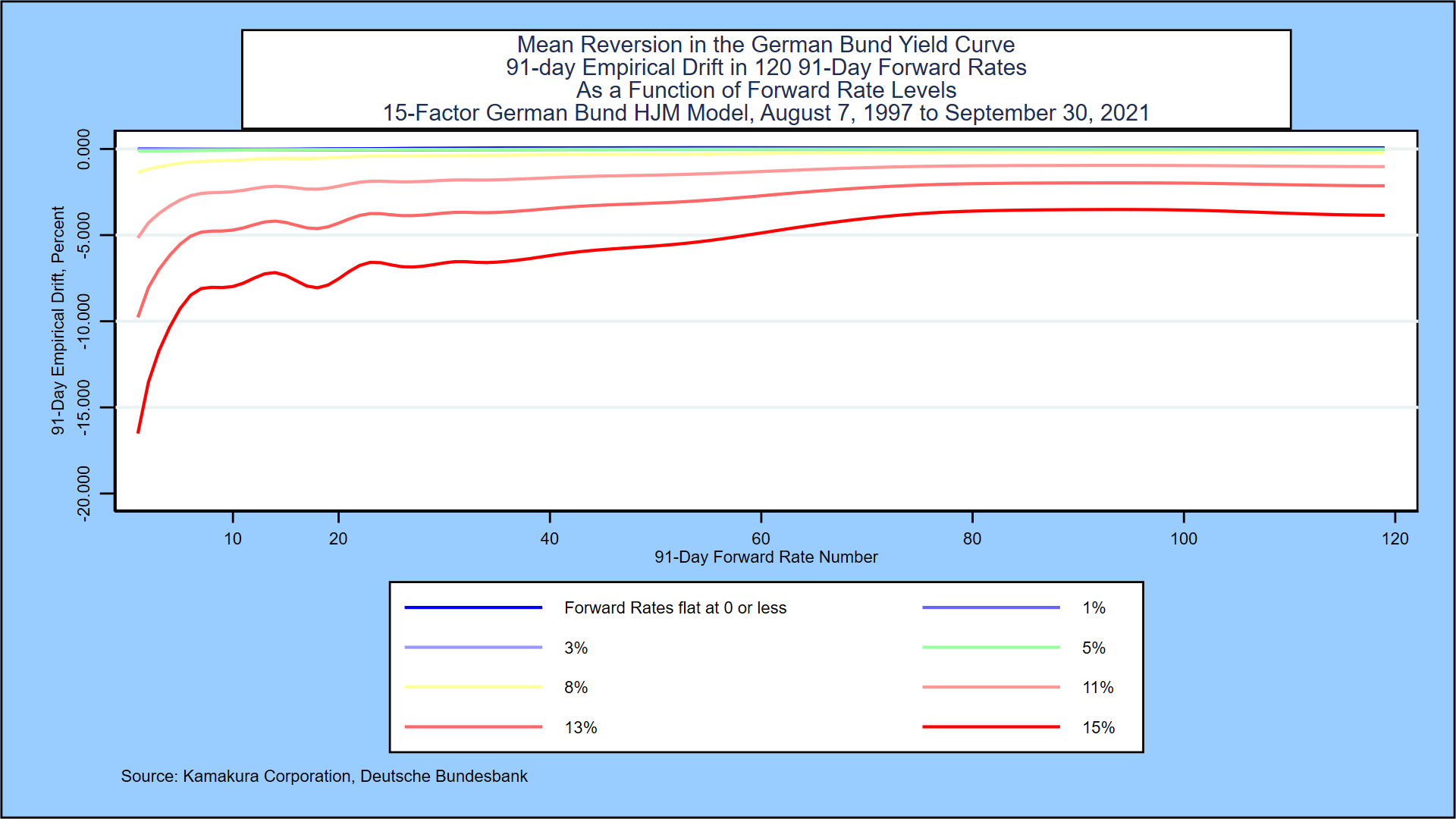The empirical drift for the World model through September 30, 2021 is shown in Exhibit B2.

Exhibit B2Simply by visual inspection, it is obvious that the fitted drift in empirical rates for the World model shows a more robust version of mean reversion: rates drift down across the 30-year term structure when yields are high.  Conversely, rates drift up when yields are low. From a Bayesian perspective, the World model’s empirical drift is more consistent with our scientific knowledge of monetary economics and finance than the empirical drift based on a “Germany only” term structure model.

We can apply the same tests to the interest rate volatility functions to confirm their robustness and that they do not “blow up” at either very high or very low-rate levels.  The first factor in the model above is the idiosyncratic movement in the first random forward rate segment.  We expect this first factor to have volatility that declines with maturity and which is higher when rates are high.  The graph below shows that those expectations are not that obvious in German Bund data:

Exhibit B3We again compare the German movements in interest rate volatility for the first factor with World experience, shown in the following graph:

Exhibit B4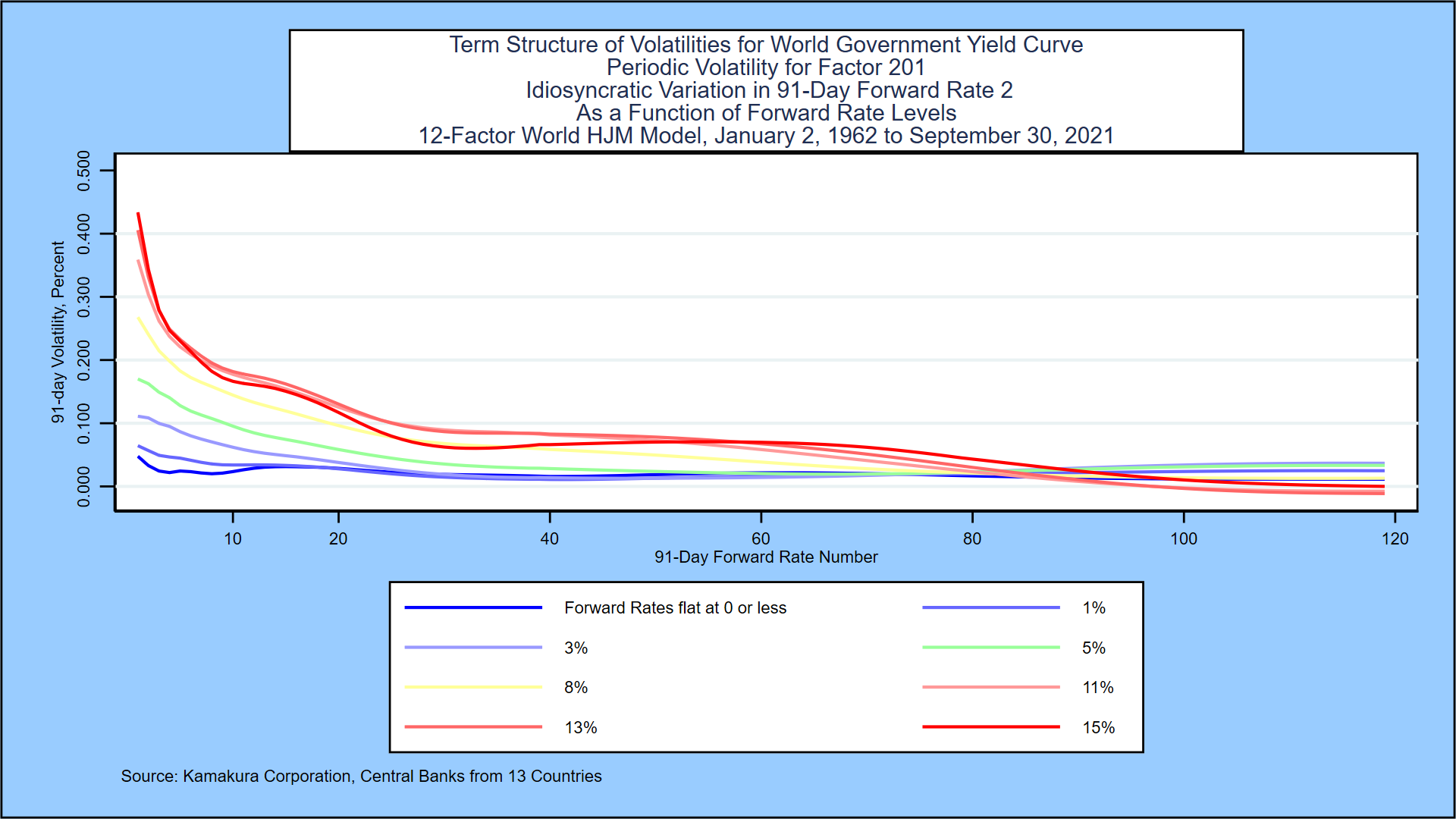The World interest rate volatility shows a dramatic difference versus the Germany-only model.  Since idiosyncratic movements in the short rate define the first factor, it makes sense that volatility of the short rate rises when rates are high and is closer to zero when rates are low.  On the long end of the curve, volatility actually changes signs, pushing the long end of the curve lower when the short rate spikes up.  This is a volatility-based indicator of mean reversion.  Again, we conclude that the World volatility function is more consistent with our knowledge of interest rate movements.

The second factor in the model is the idiosyncratic variation in the 91-day forward rate segment maturing in 30 years.  Because this point is on the right-hand side of the yield curve in most countries, we expect volatility functions can be both positive and negative.  Our main concern is that volatility does not blow up as we stress test the level of the forward rate curve.  Again, our expectations are met, as shown below.

Exhibit B5How does this compare with volatility for the second factor (which is the idiosyncratic movements in the 91-day forward rate maturing in 10 years) for the World model?  The answer is given in the graph below:

Exhibit B6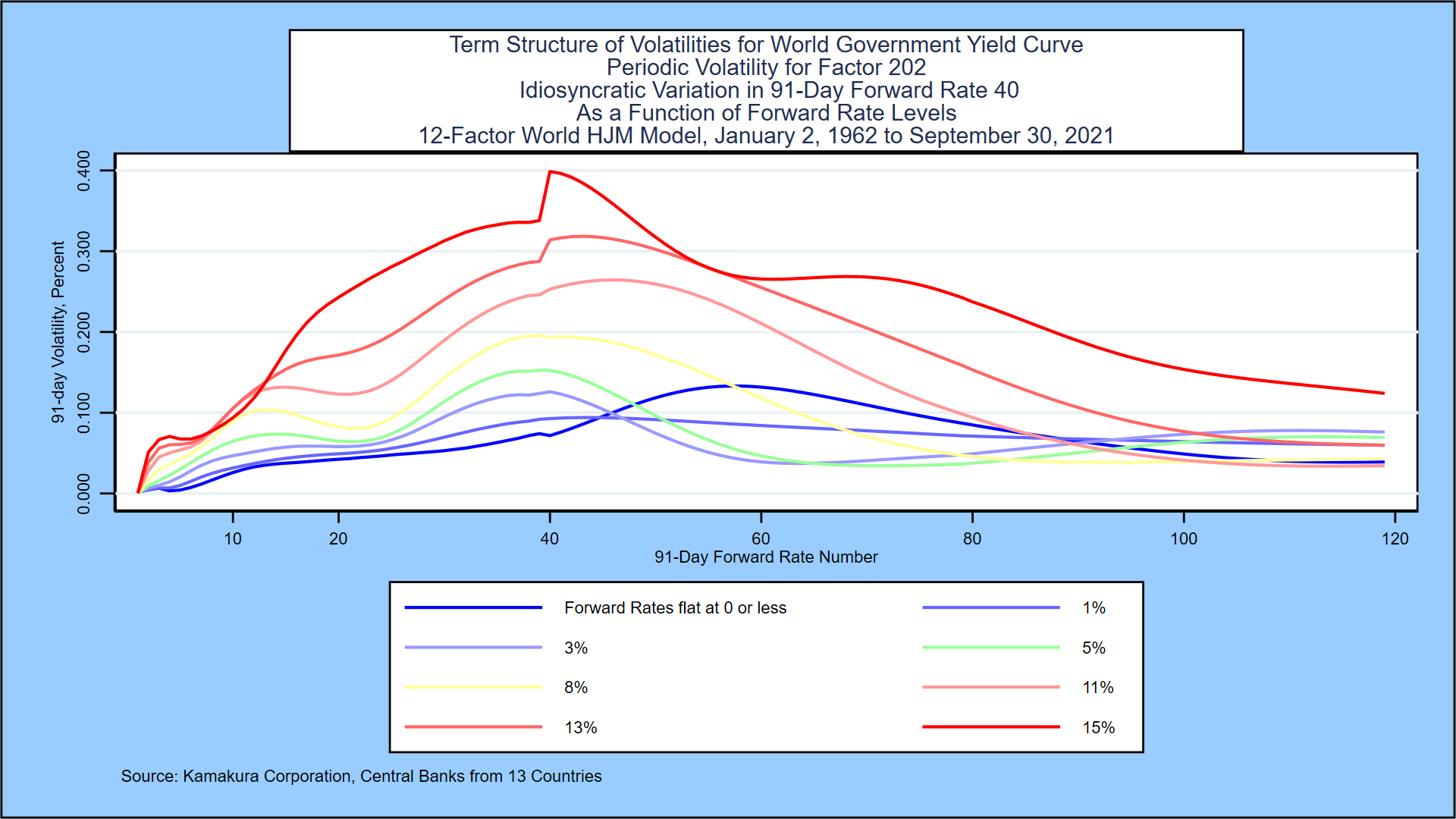Again, the results of the World model differ from the Germany-only model, but this is partly due to where the factor lies on the yield curve (at 30 years for Germany and 10 years for the World model).  An upward shock in the 10-year forward rate drives up yields in the middle of the World yield curve, with smaller impacts on the short end and long end of the curve.  Generally speaking, volatility increases as the level of the 10-year forward rate rises.  Both models show realistic shifts in volatility but at different points on the yield curve.

A forward-looking simulation using both models from the same initial Germany Bund yield curve is another way to make these differences starkly apparent.  Kamakura Corporation does such comparisons constantly and would be pleased to provide additional detail in this regard upon request to info@kamakuraco.com.

REFERENCES

Adams, Kenneth J., and Donald R. van Deventer, “Fitting Yield Curves and Forward Rate Curves with Maximum Smoothness,” Journal of Fixed Income, 1994, pp. 52-61.

Adrian, Tobias, Richard K. Crump and Emanuel Moench, “Pricing the Term Structure with Linear Regressions,” Federal Reserve Bank of New York, Staff Report 340, August 2008, revised August 2013.

Angrist, Joshua D. and Jorn-Steffen Pischke, Mostly Harmless Econometrics: An Empiricist’s Companion, Princeton University Press, Princeton, 2009.

Berger, James O. Statistical Decision Theory and Bayesian Analysis, second edition, Springer-Verlag, 1985.

Berry, Donald A. Statistics: A Bayesian Perspective, Wadsworth Publishing Company, 1996.

Campbell, John Y, Andrew W. Lo, and A. Craig McKinley, The Econometrics of Financial Markets, Princeton University Press, 1997.

Gelman, Andrew and John B. Carlin, Hal S. Stern, David B. Dunson, Aki Vehtari, and Donald B. Rubin, Bayesian Data Analysis, third edition, CRC Press, 2013.

Goldberger, Arthur S. A Course in Econometrics, Harvard University Press, 1991.

Hamilton, James D. Times Series Analysis, Princeton University Press, 1994.

Hansen, Bruce E. Econometrics, University of Wisconsin, January 15, 2015.

Hardin, James W. and Joseph M. Hilbe, Generalized Linear Models and Extensions, fourth edition, Stata Press, College Station, Texas, 2018.

Hastie, Trevor, Robert Tibshirani and Jerome Friedman, Elements of Statistical Learning: Data Mining, Inference and Prediction, Springer, second edition, tenth printing, 2013.

Heath, David, Robert A. Jarrow, and Andrew Morton, “Bond Pricing and the Term Structure of Interest Rates: A Discrete Time Approach,” Journal of Financial and Quantitative Analysis, 1990, pp. 419-440.

Heath, David, Robert A. Jarrow, and Andrew Morton, “Contingent Claims Valuation with a Random Evolution of Interest Rates,” The Review of Futures Markets, 9 (1), 1990, pp. 54 -76.

Heath, David, Robert A. Jarrow, and Andrew Morton,” Bond Pricing and the Term Structure of Interest Rates: A New Methodology for Contingent Claim Valuation,” Econometrica, 60(1),1992, pp. 77-105.

Heath, David, Robert A. Jarrow, and Andrew Morton, “Easier Done than Said”, RISK Magazine, October 1992.

Jarrow, Robert A. Modeling Fixed Income Securities and Interest Rate Options, second edition, Stanford University Press, Stanford, 2002.

Jarrow, Robert A. and Stuart Turnbull, Derivative Securities, second edition, South-Western College Publishing, Cincinnati, 2000.

Jarrow, Robert A., and Donald R. van Deventer, “Monte Carlo Simulation in a Multi-Factor Heath, Jarrow and Morton Term Structure Model,” Kamakura Corporation Technical Guide, Version 4.0, June 16, 2015

Jarrow, Robert A. and Donald R. van Deventer, “Parameter Estimation for Heath, Jarrow and Morton Term Structure Models,” Kamakura Corporation Technical Guide, Version 4.0, May 5, 2017.

Johnston, J. Econometric Methods, McGraw-Hill, 1972

Kim, Don H., and Jonathan H. Wright, “An Arbitrage-Free Three Factor Term Structure Model and the Recent Behavior of Long-Term Yields and Distant-Horizon Forward Rates,” Finance and Economics Discussion Series, Federal Reserve Board, 2005-33.

Maddala, G. S. Introduction to Econometrics, third edition, John Wiley & Sons, 2005.

Papke, Leslie E. and Jeffrey M. Wooldridge, “Econometric Methods for Fractional Response Variables with an Application to 401(K) Plan Participation Rates,” Journal of Applied Econometrics, Volume 11, 619-632, 1996.

Robert, Christian P. The Bayesian Choice: From Decision-Theoretic Foundations to Computational Implementation, second edition, Springer Science+Business Media LLC, 2007.

Stock, James H. and Mark W. Watson, Introduction to Econometrics, third edition, Pearson/Addison Wesley, 2015.

Studenmund, A. H. Using Econometrics: A Practical Guide, Addison-Wesley Educational Publishers, 1997.

Theil, Henri. Principles of Econometrics, John Wiley & Sons, 1971.

van Deventer, Donald R.  “Interest Rate Risk: Lessons from 2 Decades of Low Interest Rates in Japan,” Kamakura Corporation working paper, www.kamakuraco.com, August 11, 2016.

van Deventer, Donald R.  “A Multi-Factor Heath Jarrow and Morton Model of the United Kingdom Government Bond Yield Curve,” Kamakura Corporation working paper, www.kamakuraco.com, August 17, 2015.

van Deventer, Donald R.  “A Multi-Factor Heath Jarrow and Morton Model of the German Bund Yield Curve,” Kamakura Corporation working paper, www.kamakuraco.com, August 21, 2015.

van Deventer, Donald R.  “A Multi-Factor Heath Jarrow and Morton Model of the Australia Commonwealth Government Securities Yield Curve,” Kamakura Corporation working paper, www.kamakuraco.com, August 27, 2015.

van Deventer, Donald R. “A Multi-Factor Heath Jarrow and Morton Model of the Swedish Government Bond Yield Curve,” Kamakura Corporation working paper, www.kamakuraco.com, September 3, 2015.

van Deventer, Donald R. “Spanish Government Bond Yields: A Multi-Factor Heath Jarrow and Morton Model,” Kamakura Corporation working paper, www.kamakuraco.com, September 10, 2015.

van Deventer, Donald R. “Singapore Government Securities Yields: A Multi-Factor Heath Jarrow and Morton Model,” Kamakura Corporation working paper, www.kamakuraco.com, September 22, 2015.

van Deventer, Donald R. “The Regime Change Term Structure Model: A Simple Model Validation Approach,” Kamakura Corporation working paper, www.kamakuraco.com, January 26, 2016.

van Deventer, Donald R.  “An 8 Factor Heath, Jarrow and Morton Model for the Japanese Government Bond Yield Curve, 1974 to 2016: The Impact of Negative Rates and Smoothing Issues,” Kamakura Corporation working paper, www.kamakuraco.com, June 21, 2017.

van Deventer, Donald R. “A 10 Factor Heath, Jarrow, and Morton Stochastic Volatility Model for the U.S. Treasury Yield Curve, Using Daily Data from January 1, 1962 through June 30, 2021.” Kamakura Corporation working paper, www.kamakuraco.com, July 19, 2021.

van Deventer, Donald R. “A 7-Factor Heath, Jarrow and Morton Stochastic Volatility Model for the Government of Canada Yield Curve, Using Daily Data from January 2, 2001 to August 31, 2021.” Kamakura Corporation working paper, www.kamakuraco.com, September 22, 2021.

van Deventer, Donald R. “An 11-Factor Heath, Jarrow, and Morton Stochastic Volatility Model for the Government of Russia Yield Curve Using Daily Data from January 4, 2003 through August 31, 2021,” Kamakura Corporation working paper,  www.kamakuraco.com, September 30, 2021.

van Deventer, Donald R., Kenji Imai and Mark Mesler, Advanced Financial Risk Management, second edition, John Wiley & Sons, Singapore, 2013.

Woolridge, Jeffrey M. Econometric Analysis of Cross Section and Panel Data, The MIT Press, 2002.

Footnotes

 Kamakura Corporation, 2222 Kalakaua Avenue, Suite 1400, Honolulu, Hawaii, USA, 96815. E-Mail dvandeventer@kamakuraco.com. The author wishes to thank Prof. Robert A. Jarrow for 27 years of conversations on this topic.  The author is grateful to Daniel Dickler, Dr. Xiaoming Wang, and Theodore Spradlin for analytical and data-related assistance. The author also wishes to thank the participants at seminars organized by the Bank of Japan and the Federal Reserve Bank of San Francisco at which a paper addressing similar issues in a Japan and U.S. government bond context was presented.

 This constraint is one method for imposing the cap in stochastic volatilities suggested by Heath, Jarrow, and Morton [Econometrica, 1992] to prevent a positive possibility of (a) infinitely high rates or (more practically) (b) unrealistically high rates.

 We make this assumption while waiting for more data on negative rates to accumulate.  At the appropriate time, a more interesting functional form will be employed.

 Gelman et all , page 3.